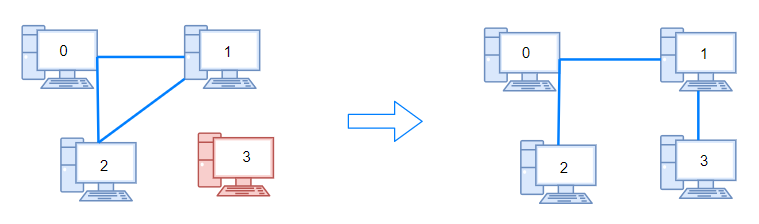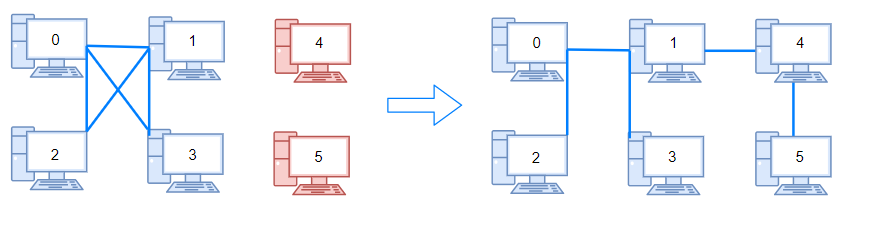1319. Number of Operations to Make Network Connected

There are `n` computers numbered from `0` to `n-1` connected by ethernet cables `connections` forming a network where `connections[i] = [a, b]` represents a connection between computers `a` and `b`. Any computer can reach any other computer directly or indirectly through the network.

Given an initial computer network `connections`. You can extract certain cables between two directly connected computers, and place them between any pair of disconnected computers to make them directly connected. Return the minimum number of times you need to do this in order to make all the computers connected. If it's not possible, return -1.

Example 1:```Input: n = 4, connections = [[0,1],[0,2],[1,2]]
Output: 1
Explanation: Remove cable between computer 1 and 2 and place between computers 1 and 3.
```

Example 2:```Input: n = 6, connections = [[0,1],[0,2],[0,3],[1,2],[1,3]]
Output: 2
```

Example 3:

```Input: n = 6, connections = [[0,1],[0,2],[0,3],[1,2]]
Output: -1
Explanation: There are not enough cables.
```

Example 4:

```Input: n = 5, connections = [[0,1],[0,2],[3,4],[2,3]]
Output: 0
```

Constraints:

• `1 <= n <= 10^5`
• `1 <= connections.length <= min(n*(n-1)/2, 10^5)`
• `connections[i].length == 2`
• `0 <= connections[i], connections[i] < n`
• `connections[i] != connections[i]`
• There are no repeated connections.
• No two computers are connected by more than one cable.

1319. Number of Operations to Make Network Connected
``````struct Solution;

struct UnionFind {
parent: Vec<usize>,
n: usize,
}

impl UnionFind {
fn new(n: usize) -> Self {
let parent = (0..n).collect();
UnionFind { parent, n }
}
fn find(&mut self, i: usize) -> usize {
let j = self.parent[i];
if i == j {
i
} else {
let k = self.find(j);
self.parent[i] = k;
k
}
}

fn union(&mut self, i: usize, j: usize) {
let i = self.find(i);
let j = self.find(j);
if i != j {
self.parent[i] = j;
self.n -= 1;
}
}
}

impl Solution {
fn make_connected(n: i32, connections: Vec<Vec<i32>>) -> i32 {
let n = n as usize;
let m = connections.len();
if m + 1 < n {
return -1;
}
let mut uf = UnionFind::new(n);
for connection in connections {
let i = connection as usize;
let j = connection as usize;
uf.union(i, j);
}
(uf.n - 1) as i32
}
}

#[test]
fn test() {
let n = 4;
let connections = vec_vec_i32![[0, 1], [0, 2], [1, 2]];
let res = 1;
assert_eq!(Solution::make_connected(n, connections), res);
let n = 6;
let connections = vec_vec_i32![[0, 1], [0, 2], [0, 3], [1, 2], [1, 3]];
let res = 2;
assert_eq!(Solution::make_connected(n, connections), res);
let n = 6;
let connections = vec_vec_i32![[0, 1], [0, 2], [0, 3], [1, 2]];
let res = -1;
assert_eq!(Solution::make_connected(n, connections), res);
let n = 5;
let connections = vec_vec_i32![[0, 1], [0, 2], [3, 4], [2, 3]];
let res = 0;
assert_eq!(Solution::make_connected(n, connections), res);
}
``````